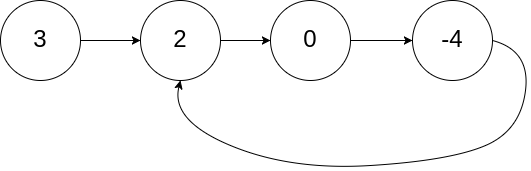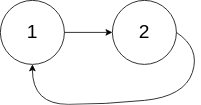### LeetCode• ㊗️
• 大家
• offer
• 多多！

## Problem

Given a linked list, return the node where the cycle begins. If there is no cycle, return null.

To represent a cycle in the given linked list, we use an integer pos which represents the position (0-indexed) in the linked list where tail connects to. If pos is -1, then there is no cycle in the linked list.

Note: Do not modify the linked list.

Example 1:

Input: head = [3,2,0,-4], pos = 1
Output: tail connects to node index 1
Explanation: There is a cycle in the linked list, where tail connects to the second node.Example 2:

Input: head = [1,2], pos = 0
Output: tail connects to node index 0
Explanation: There is a cycle in the linked list, where tail connects to the first node.Example 3:

Input: head = , pos = -1
Output: no cycle
Explanation: There is no cycle in the linked list.Follow-up: Can you solve it without using extra space?

## Code

class Solution {

while(fast != null && fast.next != null){
slow = slow.next;
fast = fast.next.next;
if(slow == fast){
break;
}
}

if(fast == null || fast.next == null) return null;

while(slow != fast){
fast = fast.next;
slow = slow.next;
}

return slow;
}
}


class Solution {
ListNode dummy = new ListNode(0);

ListNode slow = dummy;
ListNode fast = dummy;

while(true){
if(fast == null || fast.next == null) break;
slow = slow.next;
fast = fast.next.next;
if(slow == fast){
break;
}
}

if(fast == null || fast.next == null) return null;

fast = dummy;
while(true){
fast = fast.next;
slow = slow.next;
if(slow == fast){
break;
}
}

return slow;
}
}